# Bending of a BeamThis test lets you check analysis results for the bending of a beam, in the context of a static case. You will use 3D meshes.Reference:

SFM, Afnor Technique, Guide de validation des prologiciels de calcul de structures, pp124-125

##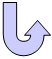Specifications

### Geometry Specifications

 CH = L = 6m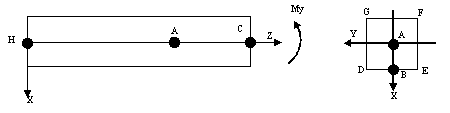AH = L' = (2/3) L DE = GF = 2a = 2m EF = DG = 2b = 2m

### Analysis Specifications

 Young Modulus (material):  E = 2e+005 GPa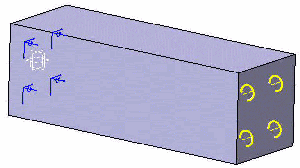Poisson's Ratio (material): ν = 0.3 Mesh Specifications: 3D elements of 500mm Restraints (User-defined): Tx = Ty = Tz = Rz = 0 on H Loads (Moment): On C, My = 4/3e+007 Nm

##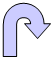Results

• The analytical solution is:• The table below presents the analysis results.
The results correspond to the means of the stress and the displacements in the section A.
Normalized results (computed results divided by analytical solution) are listed.

 Localization Type of Values Analytical Solution Values Linear Tetrahedron (TE4) Parabolic Tetrahedron (TE10) Linear Hexahedron (HE8) Parabolic Hexahedron (HE20)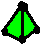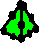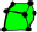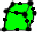Computed Results Normalized Results Computed Results Normalized Results Computed Results Normalized Results Computed Results Normalized Results Section A σzz[Mpa] 10 7.6 0.76 10 1 9.97 0.997 9.99 0.99 A UA [mm] -0.4 -0.329 0.823 -0.388 0.97 -0.389 0.973 -0.39 0.975 B WB [mm] 0.2 0.17 0.85 0.197 0.985 0.197 0.985 0.197 0.985 F or G VF = -VG [mm] 0.015 0.012 0.8 0.01485 0.99 0.0149 0.993 0.0149 0.993 D or E VD = -VE [mm] -0.015 -0.012 0.8 -0.01485 0.99 -0.0149 0.993 -0.0149 0.993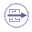To Perform the Test:

The Bending_of_a_beamTET10.CATAnalysis document presents a complete analysis of this case, computed with a mesh formed of parabolic tetrahedron elements (TE10).

The Bending_of_a_beamHE20.CATAnalysis document presents a complete analysis of this case, computed with a mesh formed of parabolic hexahedron elements (HE20).

To compute the case with linear tetrahedron elements (TE4) and linear hexahedron elements (HE8), proceed as follow:

1. Open one of the CATAnalysis documents.

2. Enter the Advanced Meshing Tools workbench.

3. In the specification tree, double-click on the mesh:

 Volume mesh  for linear tetrahedron mesh (TE4), Surface mesh for linear hexahedron mesh (HE8).

The Global Parameters dialog box appears.

4. Select the Linear element type.

5. Compute the case in the Generative Structural Analysis workbench.